By going through these CBSE Class 12 Maths Notes Chapter 7 Integrals, students can recall all the concepts quickly.

## Integrals Notes Class 12 Maths Chapter 7

→ Integration is the inverse process of differentiation. If we are given the derivative of a function and we have to find the function whose derivative is given, the process of finding the primitive or the original function is called the integration or anti-differentation.

Let $$\frac{d}{d x}$$[F(x) + c] = F ‘(x) = f(x)
⇒ F(x) + c is the antiderivative or integal of f(x). This may be written as ∫f(x)dx = F(x) + c,
where c is an arbitrary constant called constant of integration.
∫f(x) dx is called indefinite integral.

Properties of Indefinite Integral.

1. The processes of differentiation and integration are inverse processes of each other, i.e.,
$$\frac{d}{d x}$$∫f(x) dx = f(x).
2. Indefinite integrals with the same derivatives belong to the same family of curves and so they are equivalent, i.e.,
in ∫f(x) dx = F(x) + c, [F(x) + c] denotes the same family of indefinite integrals of f(x).
3. ∫[f(x) + g(x)] dx = ∫f(x) dx + ∫g(x) dx.
4. ∫k f(x) dx = k ∫f(x) dx, where k is real number.
5. If k1, k2,………… kn are the real numbers, then ∫[k1 f1(x)+ k2 f2(x) +………..+ knfn(x)] dx = k1∫f1(x)dx +k2 ∫f2(x) dx + +kn∫fn(x)dx.

→ We know the formulae for the derivatives of many functions. Corresponding integrals are given below:

 Derivatives Integrals 1. $$\frac{d}{d x}\left(\frac{x^{n+1}}{n+1}\right)$$ Where n = 0, $$\frac{d}{d x}$$(x) = 1 1. ∫xn dx = $$\frac{x^{n+1}}{n+1}$$ + C ∫dx = x + C Trigonometric Functions 2. $$\frac{d}{d x}$$(sin x) = cos x 2. ∫cos x dx = sin x + C 3. $$\frac{d}{d x}$$(tan x ) = sin x 3. ∫sin x dx = – cos x + C 4. $$\frac{d}{d x}$$(- cosec x) = cosec x cot x 4. ∫sec2 x dx = tan x + C 5. $$\frac{d}{d x}$$(- cosec x) = cosec x cot x 5. ∫cosec x cot x dx = – cosec x + C 6. $$\frac{d}{d x}$$(sec x) = sec x tan x 6. ∫sec x tan x dx = sec x + C 7. $$\frac{d}{d x}$$(- cot x) = cosec2 x 7. ∫cosec2 x dx = – cot x + C Inverse Trigonometric Functions 8. $$\frac{d}{d x}$$(sin-1 x) = $$\frac{1}{\sqrt{1-x^{2}}}$$ 8. ∫$$\frac{1}{\sqrt{1-x^{2}}}$$ dx = sin-1  x + C 9. $$\frac{d}{d x}$$(- cos-1 x) = + $$\frac{1}{\sqrt{1-x^{2}}}$$ 9. ∫$$\frac{1}{\sqrt{1-x^{2}}}$$ dx = – cos-1 x + C 10. $$\frac{d}{d x}$$(tan-1 x) = $$\frac{1}{1+x^{2}}$$ 10. ∫$$\frac{1}{1+x^{2}}$$ dx = tan-1 x + C 11. $$\frac{d}{d x}$$(- cot-1 x) = $$\frac{1}{1+x^{2}}$$ 11. ∫$$\frac{1}{1+x^{2}}$$ dx = – cot-1 x + C 12. $$\frac{d}{d x}$$(sec-1 x) = $$\frac{1}{x \sqrt{x^{2}-1}}$$ 12. ∫$$\frac{1}{x \sqrt{x^{2}-1}}$$ dx = sec-1 x + C 13. $$\frac{d}{d x}$$(- cosec-1  x) = $$\frac{1}{x \sqrt{x^{2}-1}}$$ 13. ∫$$\frac{1}{x \sqrt{x^{2}-1}}$$ dx = – cosec-1 x + C Exponential Functions 14. $$\frac{d}{d x}$$ex = ex 14. ∫ex dx = ex + C 15. $$\frac{d}{d x}\left(\frac{a^{x}}{\log a}\right)$$  =ax 15. ∫ax dx = $$\frac{a^{x}}{\log a}$$ + C Logarithmic Functions 16. $$\frac{d}{d x}$$(loge x) = $$\frac{1}{x}$$ 16. ∫$$\frac{1}{x}$$ dx = loge x + C 17. $$\frac{1}{2}$$(loga x) = $$\frac{1}{x}$$loga e 17. ∫$$\frac{1}{x}$$loga e dx = loga x + C

Geometrical Interpretation of Indefinite Integral:
∫f(x) dx = F(x) + C = y (say).
y = F(x) + C represents a family of curves. By giving different values to C, we get different members of family. These members can be obtained by shifting any of the curves parallel to itself.

Comparison between Differentiation and Integration:

 Differentiation Integration 1. It is an operation on function. 1. It is an operation on function. 2. \begin{aligned}\frac{d}{d x}\left[k_{1} f_{1}(x)+k_{2} f_{2}(x)+\ldots\right.\\\left.+k_{n} f_{n}(x)\right]\end{aligned} = k1 $$\frac{d}{d x}$$f1 (x) + k2 $$\frac{d}{d x}$$f2(x) + ……….+ kn $$\frac{d}{d x}$$fn (x) 2. ∫ k1 $$\frac{d}{d x}$$f1 (x) + k2 $$\frac{d}{d x}$$f2 (x) + ……….+ kn $$\frac{d}{d x}$$fn (x) = k1∫f21(x) dx + k2∫f2 (x) dx + ……… + kn∫fn (x) dx 3. Some functions are not differentiable. 3. All functions are not integrable. 4. The derivative of a function, if it exists, is unique. 4. The integral of a function is not unique. 5. ¡f a polynomial function of a degree n is differentiated, we obtain a polynomial of degree n – 1. 5. If a polynomial function of a degree n is integrated, we get a polynomial of degree n + 1. 6. We can obtain a derivative at a point. 6. Integral of a function may be obtained over an interval in which f is defined. 7. Slope of tangent at a point x = x1 is f ‘(x1). 7. Integral of a function represents a family of curves. 8. If the distance traversed at any time f is known, we can find velocity and acceleration. 8. When the velocity or acceleration at any time t is known, we can find the distance traversed in time t, 9. Differentiation is a process involving limits. 9. Integration too involves limits.

Integration by Substitution:
Let I = ∫f(x) dx. This integral can be transformed into another form by changing the independent variable x to t by putting x=g(t).
∴ $$\frac{dx}{dt}$$ = g'(t) or dx = g'(t) dt
∴ I = ∫f[g(t)]g'(t) dt

Note: While making a substitution, it should be kept in mind that the f[g(t)] is in the form of some standard formula, whereas g'(t) is a factor, along with f[g(t)] e.g.
Consider the integral I = ∫x2 cos(x3 + 2)dx
If we put x3 + 2 = t, its derivative 3x2 is a factor and cos t can easily be integrated.Some Results:

1. ∫tan x = log|sec x| + C
2. ∫cot x = log|sin x| + C
3. ∫sec x = log|sec x + tan x| + C
4. ∫cosec x dx = log|cosec x – cot x| + C

→ Use of Trigonometric Identities:
Use following trigonometric identities for integrating the functions such as sin2x.cos2x, sin3x, cos3x, sin x cos x, etc.

1. sin2 x = $$\frac{1-\cos 2 x}{2}$$, cos2 x = $$\frac{1+\cos 2 x}{2}$$
2. sin3 x = $$\frac{3 \sin x-\sin 3 x}{4}$$, cos2 x = $$\frac{3 \cos x+\cos 3 x}{4}$$
3. 2sinA cosB = sin(A + B) + sin(A – B)
2cosA sinB = sin(A + B) – sin(A – B)
2cosA cosB = cos(A + B) + cos(A – B)
2sinA sinB = cos(A – B) – cos(A + B)

Some more Integrals: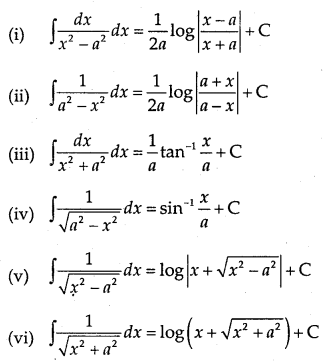→ How to integrate when the integral has ax2 + bx + c or $$\sqrt{a x^{2}+b x+c}$$ in the denominator?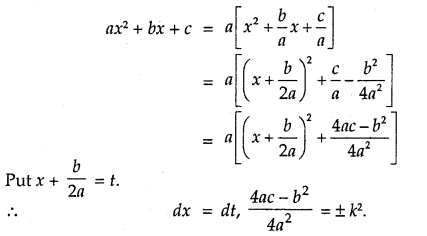So, ax2 + bx + c changes to t2 ± k2.
Thus, $$\frac{1}{a x^{2}+b x+c}$$ converts into the form $$\frac{1}{t^{2} \pm k^{2}}$$ and
$$\frac{1}{\sqrt{a x^{2}+b x+c}}$$ converts into the form $$\frac{1}{\sqrt{t^{2} \pm \dot{k}^{2}}}$$.

→ To find the integration of type ∫$$\frac{p x+q}{a x^{2}+b x+c}$$ dx or ∫$$\frac{p x+q}{\sqrt{a x^{2}+b x+c}}$$ dx:
Put px + q = A$$\frac{d}{d x}$$(ax2 + bx + c) + B

Compare the two sides and find the value of A and B.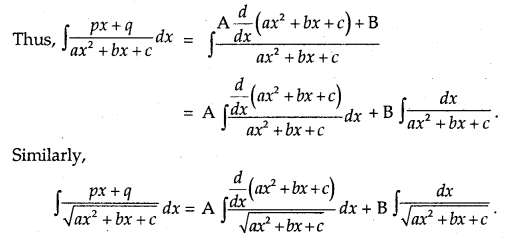Partial Fractions of Rational Functions.
(a) Let $$\frac{\mathrm{P}(x)}{\mathrm{Q}(x)}$$ be a rational function, where P(x) and Q(x) are
polynomials, where Q(x) ≠ 0.

1. If degree of P(x) is less than degree of Q(x), then $$\frac{\mathrm{P}(x)}{\mathrm{Q}(x)}$$ called a proper rational function.
2. If degree of P(x) is greater than the degree of Q(x), then $$\frac{\mathrm{P}(x)}{\mathrm{Q}(x)}$$ is known as an improper rational function.

This can be expressed in the form f(x) + $$\frac{\mathrm{P}_{1}(x)}{\mathrm{Q}(x)}$$ by dividing P(x) by Q(x), so that degree of P1(x) is less than the degree of Q(x).

(b) 1. Let $$\frac{\mathrm{P}(x)}{\mathrm{Q}(x)}$$ be a proper rational function. Find the factors of Q(x). Let these factors be linear.
P(x)
Suppose Q(x) = (x + a)(x + b)(x + c), Then, $$\frac{\mathrm{P}(x)}{\mathrm{Q}(x)}$$ is written as
$$\frac{\mathrm{P}(x)}{\mathrm{Q}(x)}=\frac{\mathrm{A}}{x+a}+\frac{\mathrm{B}}{x+b}+\frac{\mathrm{C}}{x+c}$$
or
P(x) = A(x + b)(x + c) + B(x + a)(x + e) + C(x+a)(x+ b)

It is an identity which is true for all values of x ∈ R.
To find A,put x =-a.
To find B, put x = – b.
To find C, put x = – c.
Thus, the fractions so obtained on the R.H.S. are the partial fractions. e.g.
Let us find the partial fraction of $$\frac{1}{(x-1)(x-2)}$$

∴ $$\frac{1}{(x-1)(x-2)}=\frac{A}{x-1}+\frac{B}{x-2}$$
1 = A(x – 2) + B(x – 1)
Put x = 1, 1 = A(1 – 2) = – A
∴ A = -1

Put x = 2, 1 = B(2 – 1) = B
∴ B = 1

$$\frac{1}{(x-1)(x-2)}=\frac{-1}{x-1}+\frac{1}{x-2}$$

2. Let the linear factor(s) be repeated. Q(x) = (x + a)(x + b)2.
Then,
$$\frac{\mathrm{P}(x)}{\mathrm{Q}(x)}=\frac{\mathrm{A}}{x+a}+\frac{\mathrm{B}}{x+b}+\frac{\mathrm{C}}{(x+b)^{2}}$$
Also, P(x) = A(x + b)2 + B(x + n)(x + b) + C(x + a)

3. Suppose one of the factors of Q(x) be quadratic.
Let Q(x) = (x + a)(x2 + bx + c). Then,
$$\frac{\mathrm{P}(x)}{\mathrm{Q}(x)}=\frac{\mathrm{A}}{x+a}+\frac{\mathrm{B} x+\mathrm{C}}{x^{2}+b x+c}$$
Also, P(x) A(x2 + b + c) ÷ (x + a)(Bx + c)
Put x = – a, value of A is obtained.
Put x =0, 1, -1, etc., and obtain equations involving A, B, C.

Substitute the value of A in these equations. Then, solve them to find the values of B and C.
OR
Compare the co-efficients of x2, x and constant. Solve the equations so obtained e.g.: Let us find the partial fractions of
$$\frac{1}{(x-1)\left(x^{2}+1\right)}$$
$$\frac{1}{(x-1)\left(x^{2}+1\right)}=\frac{A}{x-1}+\frac{B x+C}{x^{2}+1}$$
∴ 1 = A(x2 + 1) + (x – 1)(Bx + C)
1 = A(x2 + 1) + B(x2 – x) + C(x – 1)
Put x=1, I =A(1 + 1) = 2A
∴ A = $$\frac{1}{2}$$.

Comparing the coefficients of x2, we get
O = A + B
∴ B = -A = – $$\frac{1}{2}$$

Comparing the coefficients of x, we get
O = -B + C
∴ C = B = –$$\frac{1}{2}$$.
∴ $$\frac{1}{(x-1)\left(x^{2}+1\right)}=\frac{1}{2(x-1)}-\frac{x+1}{2\left(x^{2}+1\right)}$$.

Note: It is obvious that by converting the rational function into partial fractions, we can easily integrate the given rational function.

Integration by Parts
Let u and v be the functions of x, then
∫uv dx = u∫v dx – ∫u'[∫vdx]dx
= u × Integral of v – Integral of (derivative of u × Integral of v]

Note: Out of two functions, which function is to be considered as first. Usually, we proceed as follows:
∫xn f(x) dx xn = u is First function.
∫(Inverse trig, function) × f(x) dx

Inverse trig. function = u is First function.
∫(log x)f(x) dx, Take log x = u as first function.

Some Integrals: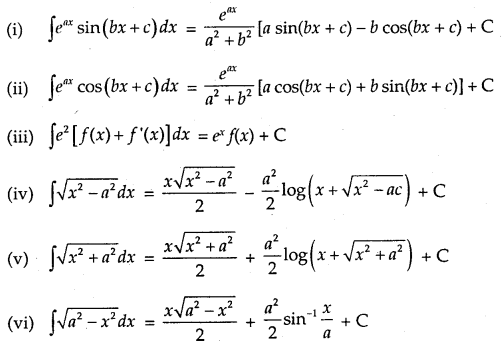Definite Integral as the Limit of Sum
Definite integral as a limit of a sum is defined aswhere h = $$\frac{b-a}{n}$$. As h → 0, n → ∞.

Note: Some useful series to find the definite integral as the limit of the sum.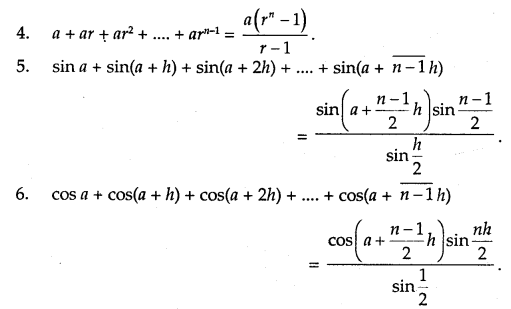Area Function:
abf(x) dx is defined as the area of the region bounded by the curve y = f(x), a ≤ x ≤ b,the x-axis and the ordinates x = a and x = b. Let x be a given point in [a, b]. Then,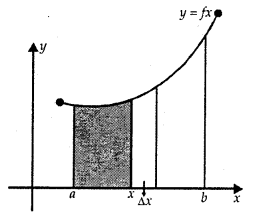ab f(x) dx represents the area of the shaded region. It is as shmmed that f(x) > 0 for x ∈ [a, b].
This function is denoted by A(x) which is known as area function. i.e.,
A(x) = ∫ax f(x) dx.

→ First Fundamental Theorem of Integral Calculus
Let f be a continuous function on the closed interval [a, b] and let A(x) be the area function. Then, A'(x) =f(x) for all x E [a, b].

→ Second Fundamental Theorem of Integral Calculus
Let f be a continuous function defined on closed interval [a, b] and F be antiderivative of f Then,
∫a b f(x) = [F(x)]ba = F(b) — F(a).

→ Evaluation of Definite Integral by Substitution

1. To evaluate ∫a b f(x) dx, let the substitution be x = g(t) such that dx = g'(t) dt.
2. Let t = t1 at x = a, t = t2 at x = b.

The new limits are t1 to t.
∴ ∫ab f(x) dx = ∫t1 t2 f[g(t)g'(t) dt
= F(t2) – F(t1), where
∫f[g(t)]g'(t) dt = F(t).

Properties of Definite Integrals: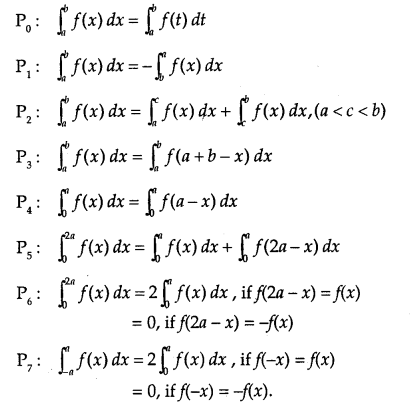1. DEFINITION
If $$\frac{d}{d x}$$ f(x) = F(x), then ∫F(x) dx = f(x) + c, where ‘c’ is a constant of integration.

2. STANDARD RESULTS

(i). Power Rule. ∫ xn dx = $$\frac{x^{n+1}}{n+1}$$ + c, provided n ≠ – 1
(ii) ∫ $$\frac{1}{x}$$ dx = log |x| + c, x ≠ 0
(iii) ∫axdx = $$\frac{a^{x}}{\log a}$$ + c, a > 0, a ≠ 1 for all x ∈ R
(iv) ∫ ex dx = ex + c ∀ x ∈ R
(v) ∫ sin x dx= -cosx+c ∀ x ∈ R
(vi) ∫ cos x dx = sin x + c ∀ x ∈ R
(vii) ∫ sec2 xdx = tan x + c, x ≠ an odd multiple of $$\frac{\pi}{2}$$
(viii) ∫ cosec2 x = – cot x + c, x ≠ an even multiple of $$\frac{\pi}{2}$$
(ix) ∫ sec x tan x dx = sec x + c, x ≠ an odd multiple of $$\frac{\pi}{2}$$
(x) ∫ cosec x cot x dx = – cosec x + c,x ≠ an even multiple of $$\frac{\pi}{2}$$
(xi) ∫ tan x dx – – log | cos x | + c = log |sec x| + c, x ≠ x an odd multiple of $$\frac{\pi}{2}$$
(xii) ∫ cot x dx = log |sin x| + c, x ≠ an even multiple of $$\frac{\pi}{2}$$
(xiii) ∫ sec x dx = log |sec x + tan x| + c, x ≠ an odd multiple of $$\frac{\pi}{2}$$
(xiv) ∫ cosec x dx =  log |cosec x – cot x| + c, x ≠ an even multiple of $$\frac{\pi}{2}$$.

3. FUNDAMENTAL THEOREMS

(i) ∫ a f(x) dx = a ∫ f(x)dx, where ‘a ’ is any real constant
(ii) ∫ [f1(x) ± f2(x)]dx = ∫ f1(x)dx ± ∫ f2(x)dx
(iii) $$\frac{d}{d x}$$[∫f(x)dx] = f(x)
(iv) ∫$$\frac{f^{\prime}(x)}{f(x)}$$dx = log |f(x)| + c
(v) ∫ f(x))n f'(x)dx = ($$\frac{(f(x))^{n+1}}{n+1}$$ + c, n ≠ -1
(vi) ∫ $$\frac{1}{\sqrt{a^{2}-x^{2}}}$$dx = sin-1$$\frac{x}{a}$$ + c
(vii) ∫ $$\frac{1}{\sqrt{a^{2}-x^{2}}}$$dx = $$\frac{1}{a} \tan ^{-1} \frac{x}{a}$$ + c
(viii) ∫ $$\frac{1}{\sqrt{a^{2}+x^{2}}}$$dx = log|x + $$\sqrt{a^{2}+x^{2}}$$|+ c
(ix) ∫ $$\frac{d x}{\sqrt{x^{2}-a^{2}}}$$ = log|x + $$\sqrt{x^{2}-a^{2}}$$ |+ c
(x) ∫ $$\sqrt{a^{2}-x^{2}} d x=\frac{x}{2} \sqrt{a^{2}-x^{2}}+\frac{a^{2}}{2} \sin ^{-1} \frac{x}{a}+c$$
(xi) ∫ $$\sqrt{a^{2}+x^{2}} d x=\frac{x}{2} \sqrt{a^{2}+x^{2}}+\frac{a^{2}}{2} \log \left|x+\sqrt{a^{2}+x^{2}}\right|+c$$
(xii) ∫ $$\sqrt{x^{2}-a^{2}} d x=\frac{x}{2} \sqrt{x^{2}-a^{2}}-\frac{a^{2}}{2} \log \left|x+\sqrt{x^{2}-a^{2}}\right|+c$$
(xiii) ∫ $$\frac{1}{a^{2}-x^{2}}$$dx = $$\frac{1}{2 a} \log \left|\frac{a+x}{a-x}\right|$$+ c
(xiv) ∫ $$\frac{1}{x^{2}-a^{2}}$$dx = $$\frac{1}{2 a} \log \left|\frac{x-a}{x+a}\right|$$+ c

4. IMPORTANT RULES

(i) Rule to integrate ∫ sinm x cosn x dx.
(a) If the index of sin x is a positive odd integer, put cos x = t.
(b) If the index of cos x is a positive odd integer, put sin x = t.

(ii) Rule to integrate : $$\int \frac{1}{a \sin ^{2} x+b \cos ^{2} x} d x, \int \frac{1}{a+b \cos ^{2} x} d x$$ ; etc.
a sin x + b cos x J a + b cos x
(a) Divide the numerator and denominator by cos2x
(b) Replace sec2x, if any, in the denominator by 1 + tan2x
(c) Put tan x = t so that sec2x dx = dt.

(iii) Integration of Parts.
Integral of the product of two functions = First function x Integral of second – Integral [(diff. coeff. of first) x (integral of second)].

(iv) Rule to integrate $$\int \frac{1}{\text { linear } \sqrt{\text { linear }}} d x$$ or $$\int \frac{1}{\text { quadratic } \sqrt{\text { linear }}} d x$$ Put $$\sqrt{\text { linear }}=t$$

(v) Rule of integrate $$\int \frac{1}{\text { linear } \sqrt{\text { quadratic }}} d x$$ Put linear = $$\frac { 1 }{ t }$$

(vi) Rule to integrate $$\int \frac{x d x}{\text { (Pure Quad.) } \sqrt{\text { Pure Quad }}}$$. Put $$\sqrt{\text { Pure Quad }}$$ = t

(vii) Rule to integrate $$\int \frac{d x}{\text { (Pure Quad.) } \sqrt{\text { Pure Quad }}}$$ Put x = $$\frac{1}{t}$$ and $$\sqrt{\text { Pure Quad }}$$ = u.

5. Integral As The Limit of a sum

$$\int_{a}^{b}$$ f(x)dx = $$\lim _{h \rightarrow 0}$$ h[f(a)+f(a + h)+f(a + 2h)+… + f(a + $$\overline{n-1}$$h)], where h = $$\frac{b-a}{n}$$.

6. FUNDAMENTAL THEOREM OF INTEGRAL CALCULUS

$$\int_{a}^{b}$$f(x) dx = F(b) – F(a), where ∫ f(x) dx = F(x).

7. PROPERTIES OF DEFINITE INTEGRALS

I. $$\int_{a}^{b}$$ f(x)dx = $$\int_{a}^{b}$$ f(t) dt

II. $$\int_{a}^{b}$$ f(x)dx = –$$\int_{a}^{a}$$f(x)dx. Particular case: $$\int_{a}^{a}$$f(x)dx = 0

III. $$\int_{a}^{b}$$ f(x)dx = $$\int_{a}^{b}$$ f(a + b – x)dx.

IV. $$\int_{a}^{b}$$ f(x)dx = $$\int_{a}^{c}$$f(x)dx + f(x)dx = $$\int_{c}^{b}$$ f(x)dx, where a < b < c

V. $$\int_{0}^{a}$$ f(x)dx = $$\int_{a}^{b}$$ f(a – x)dx

VI. $$\int_{-a}^{a}$$ f(x)dx = 0 if f(-x) = -f(x)
= 2$$\int_{0}^{a}$$ f(x)dx if f(-x) = f(x)

VII. $$\int_{0}^{2a}$$ f(x)dx = $$\int_{0}^{a}$$ f(x)dx =$$\int_{0}^{a}$$ f(2a – x)dx

VIII. $$\int_{0}^{2a}$$ f(x)dx = 2$$\int_{0}^{a}$$ f(x) dx
= 0

f(2a-x)dx = f(x)
if (2a – x) = f(x)